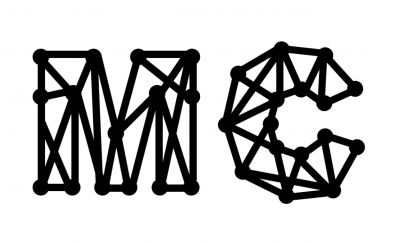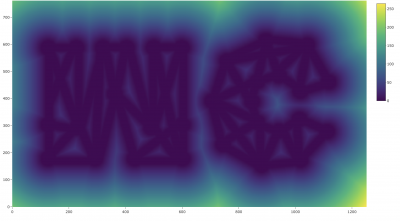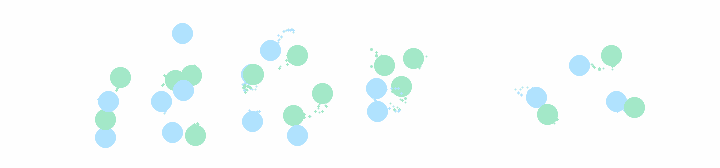# MC animated logo

Connor Fitch and Taylor Meredith

Here is the final result

Our goal was to design a logo for the REU and build a code that could animate a random walk of scattered points that would converge to our logo design.

We start from a black and white image of our logo:Each white pixel of the image is assigned a zero, while each black pixel is assigned a one. We want our animation to start from uniformly distributed black points, each following the path of a Markov chain that would bring all the points to the black pixel locations (i.e. our logo). Once the a point hits the logo, it says near it.

First we created a distance function $$d(x,y)$$ to find the distance between each point on the screen and the nearest black pixel. Below is the heat map that depicts the distance function distribution of our image. Â You may notice that the logo is flipped here because in converting the image to a matrix, the top left corner is read as the origin.We then constructed a Markov chain based on the distance function. In this Markov chain, the probability of moving to a neighboring pixel location is biased towards the set of black pixels. The transition function is

$$p(x,y) = \frace^{- \beta d(x,y)}}e^{- \beta d(x’+1,y’)}+e^{- \beta d(x’,y’+1)}+e^{- \beta d(x’-1,y’)}+e^{- \beta d(x’,y’-1)}$$

where $$(x,y)$$ is the point’s new location and $$(x’,y’)$$ is the point’s old location, and $$\beta>0$$ is a parameter representing the strengths of the bias.

The value of $$\beta$$ is small when the point is in a white pixel location, and we then switch to large $$\beta$$ once hitting a black pixel location. This change in $$\beta$$ greatly decreases the likelihood that a point would move from a black pixel location to a white pixel location, hence once a point reaches a black pixel region it should stay within the region. Within the black pixel region the distance is zero, thus $$p(x,y)$$ becomes a uniform distribution where the points can move with a constant probability.

The code was written in R and the animation was done with Plotly. Here is the raw animation:

For this logo animation, we took inspiration from Jan Krepl who built a similar animation for logos using a Markov Chain Monte Carlo method. You can check out the website here: https://jankrepl.github.io/creating-animations-with-MCMC/

Krepl designed his code to drop points over time, which is different from our code which starts with all the points scattered. Starting with this logo:Finally, here is an implementation of Krepl’s code to our logo: